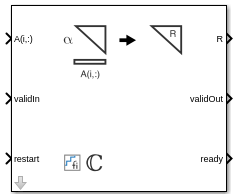# Complex Partial-Systolic Q-less QR Decomposition with Forgetting Factor

Q-less QR decomposition for complex-valued matrices with infinite number of rows

• Library:
• Fixed-Point Designer HDL Support / Matrices and Linear Algebra / Matrix Factorizations

•## Description

The Complex Partial-Systolic Q-less QR Decomposition with Forgetting Factor block uses QR decomposition to compute the economy size upper-triangular R factor of the QR decomposition A = QR, without computing Q. A is an infinitely tall complex-valued matrix representing streaming data.

When the regularization parameter is nonzero, the Complex Partial-Systolic Q-less QR Decomposition with Forgetting Factor block initializes the first upper-triangular factor R to λIn before factoring in the rows of A, where λ is the regularization parameter and In = `eye(n)`.

## Ports

### Input

expand all

Rows of matrix A, specified as a vector. A is an infinitely tall matrix of streaming data. If A is a fixed-point data type, A must be signed, use binary-point scaling, and have the same word length as B. Slope-bias representation is not supported for fixed-point data types.

Data Types: `single` | `double` | `fixed point`
Complex Number Support: Yes

Whether inputs are valid, specified as a Boolean scalar. This control signal indicates when the data at the `A(i,:)` input port is valid. When this value is 1 (`true`) and the value at `ready` is 1 (`true`), the block captures the values at the `A(i,:)` input port. When this value is 0 (`false`), the block ignores the input samples.

After sending a `true` `validIn` signal, there may be some delay before `ready` is set to `false`. To ensure all data is processed, you must wait until `ready` is set to `false` before sending another `true` `validIn` signal.

Data Types: `Boolean`

Whether to clear internal states, specified as a Boolean scalar. When this value is 1 (`true`), the block stops the current calculation and clears all internal states. When this value is 0 (`false`) and the `validIn` value is 1 (`true`), the block begins a new subframe.

Data Types: `Boolean`

### Output

expand all

Economy size QR decomposition matrix R, returned as a scalar or vector. R is an upper triangular matrix. The size of matrix R is n-by-n. R has the same data type as A.

Data Types: `single` | `double` | `fixed point`

Whether the output data is valid, specified as a Boolean scalar. This control signal indicates when the data at output port `R` is valid. When this value is 1 (`true`), the block has successfully computed the matrix R. When this value is 0 (`false`), the output data is not valid.

Data Types: `Boolean`

Whether the block is ready, returned as a Boolean scalar. This control signal indicates when the block is ready for new input data. When this value is `1` (`true`) and the `validIn` value is `1` (`true`), the block accepts input data in the next time step. When this value is `0` (`false`), the block ignores input data in the next time step.

After sending a `true` `validIn` signal, there may be some delay before `ready` is set to `false`. To ensure all data is processed, you must wait until `ready` is set to `false` before sending another `true` `validIn` signal.

Data Types: `Boolean`

## Parameters

expand all

Number of columns in input matrix A, specified as a positive integer-valued scalar.

#### Programmatic Use

 Block Parameter: `n` Type: character vector Values: positive integer-valued scalar Default: `4`

Forgetting factor applied after each row of the matrix is factored, specified as a real positive scalar. The output is updated as each row of A is input indefinitely.

#### Programmatic Use

 Block Parameter: `forgettingFactor` Type: character vector Values: positive integer-valued scalar Default: `0.99`

Regularization parameter, specified as a nonnegative scalar. Small, positive values of the regularization parameter can improve the conditioning of the problem and reduce the variance of the estimates. While biased, the reduced variance of the estimate often results in a smaller mean squared error when compared to least-squares estimates.

#### Programmatic Use

 Block Parameter: `regularizationParameter` Type: character vector Values: real nonnegative scalar Default: `0`

expand all